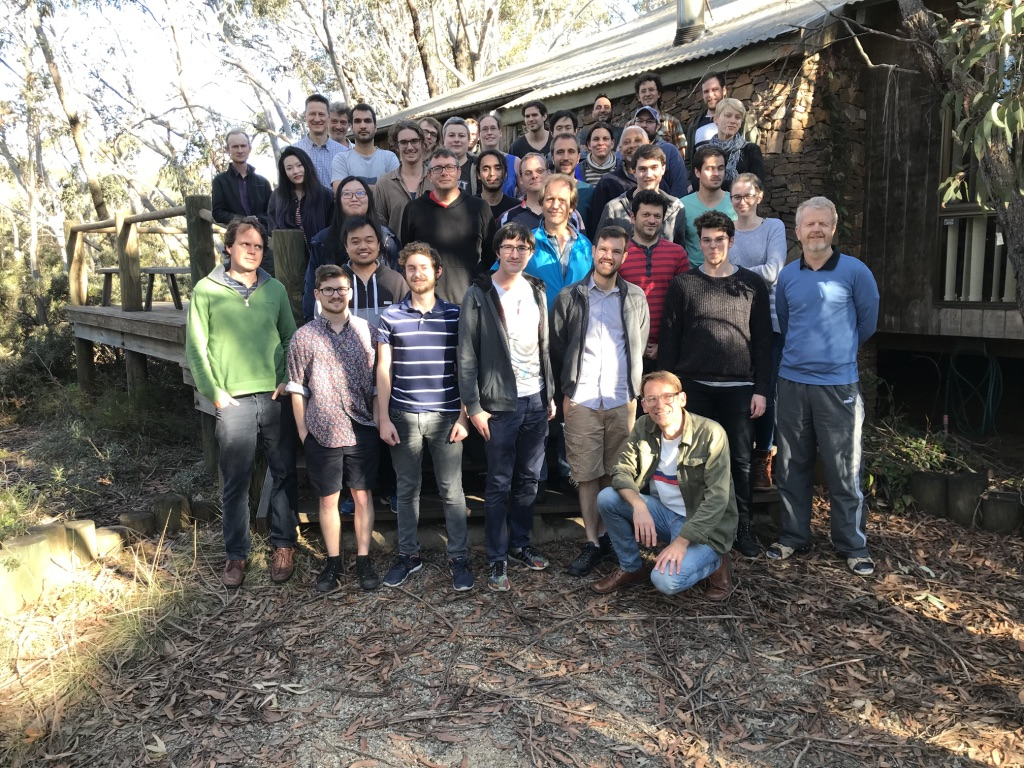SDG
2023

## Members of SDG

### Faculty

• Eduardo Altmann (USyd, Maths): Hamiltonian systems, transient chaos, extreme events, complex networks
• Holger Dullin (USyd, Maths): Hamiltonian systems, rigid body dynamics with applications in biomechanics, topology of integrable systems, semiclassical quantization, volume preserving mappings
• Chris Angstmann (UNSW, Maths): pattern formation, biomathematics, anomalous diffusion
• Sasha Fish (USyd, Maths): ergodic theory, applications of dynamics to number theory
• Gary Froyland (UNSW, Maths): smooth and numerical ergodic theory, applications to atmospheric and oceanic flows
• Dave Galloway (USyd, Maths): solar and geodynamo modelling
• Ben Goldys (USyd, Maths): stochastic partial differential equations, analysis on infinite-dimensional spaces, long time behaviour of solutions to stochastic ordinary and partial differential equations, optimal control
• Georg Gottwald (USyd, Maths): geophysical fluid dynamics, pattern formation in biological and chemical systems, nonlinear time series analysis
• Shane Keating (UNSW, Maths): mixing and stirring in geophysical turbulence, satellite altimetry, ocean state estimation, eddy parameterization
• Charlie Macaskill (USyd, Maths): geophysical fluid dynamics, especially vortex dynamics and internal waves, acoustics in complex and random media with applications in biomedical ultrasound, mathematical theory of combustion
• Robert Marangell (USyd, Maths): dynamical systems, stability of nonlinear waves
• Gary Morriss (UNSW, Physics): statistical mechanics of nonequilibrium systems
• Alina Ostafe (UNSW, Maths): arithmetic dynamical systems
• Milena Radnovic (USyd, Maths): integrable systems, billiards
• John Roberts (UNSW, Maths): discrete integrable systems, symmetry and time-reversal symmetry, arithmetic dynamics
• Martin Wechselberger (USyd, Maths): invariant manifold theory, canard theory, geometric singular perturbation theory with applications to the nervous system and cell signalling

### Postdoctoral Fellows

• Jason Atnip (UNSW, Maths): ergodic theory, random dynamical systems, statistical limit theorems
• Kamil Bulinski (UNSW, Maths): ergodic theory, combinatorial number theory
• Jae Min Lee (USyd, Maths): Geometric fluid dynamics, Hamiltonian PDEs, spectral theory
• Ian Lizarraga (USyd, Maths): geometric singular perturbation theory, slow-fast dynamical systems, coupled oscillators
• Debopriya Mukherjee (USyd and UNSW, Math): stochastic PDEs and optimal control of fluids

• Lachlan Burton (USyd, Math): transient chaos, billiards
• Nicholas Cranch (USyd, Math): machine learning for dynamical systems
• Mitch Curran (USyd, Maths): Maslov index in standing waves of the NLS equation on quantum graphs
• Sean Dawson (USyd, Maths): quantum aspects of integrable systems related to separation of variables
• Michael Denes (UNSW, Maths): finite-time coherent sets in ocean dynamics
• Zach Issa (USyd, Maths): Skorokhod embedding theorem with financial applications
• Vladimir Jakovljevic (USyd, Maths): caustics of pseudo-Euclidean integrable billiards
• Damien McLeod (USyd, Maths): Classification of degenerate classical and quantum integrable systems
• Diana Nguyen (USyd, Maths): integrable systems related to separation of variables
• Farah El Rafei (UNSW, Maths): spin waves in nanowires under stochastic perturbations
• Christopher Rock (UNSW, Maths): theory and numerics for the dynamic Laplacian
• Camelia Tiplea (USyd, Math): pricing of American options
• James Yang (USyd, Math): optimal control of slow fast systems
• Wenqi Yue (USyd, Math): model reduction for synchronization in complex networks